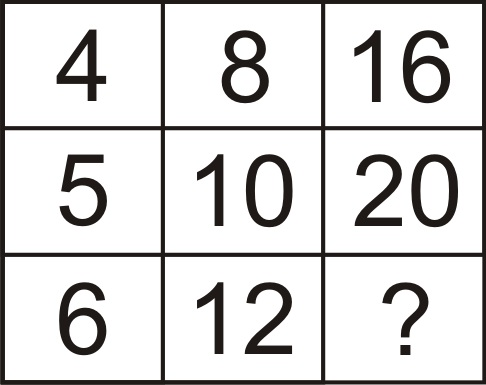Home > Math Shortcuts > Brain Trigger #10 – Sample Number puzzle question

# Brain Trigger #10 – Sample Number puzzle question

Now here we will discuss few question answers on Number puzzle which are very common in competitive exams. These Number puzzle practice question answer session will help you to prepare for your examination. Try to Solve these questions with your math skills. Apply your skills on shortcut tricks to solve these Number puzzle question.

We try our best to get all type of shortcut tricks to solve these problems and bring them together here in this website. what you need to do is to apply these tricks in Number puzzle problem to solve. These questions can be solvable without using any shortcut methods also.

Now try to solve a question on Number puzzle. What you need to do is to, read the question carefully and then try yourself to solve the question. We provide the answer below. If you solve the problem then check your answer with us. If you don’t know how to do it then check the solution below.

Every page of this section is contain a question on Number puzzle with its detail explanation. By cliking on Next/Previous link you can navigate through other questions.

Let’s starts the question and answer session. Today’s question is:

What number should replace the question mark ?To know the answer of this question please scroll down. Take as much time as you want to get the answer of this question. But try to solve this as quicker as you can.

(Scroll down to get the answer)

(Scroll down to get the answer)

(Scroll down to get the answer)

(Scroll down to get the answer)

Here is the Answer of previous question. If you try the problem and got an answer then check your solution with us. Well Done if you correctly answer the previous question, but don’t worry if you are not able to answer the question correctly. Here is the answer of the question with explanation.

You can do this way ….

4+ 4 = 8 then 8 + 8 = 16 Similarly
5 + 5 = 10 then 10 + 10 = 20
6 + 6 = 12 then 12 + 12 = 24

 << PREVIOUS QUESTION NEXT QUESTION >>

Here we provide some other questions on different topics also. Click on the Next/Previous link to get more Question Answer session.

1.